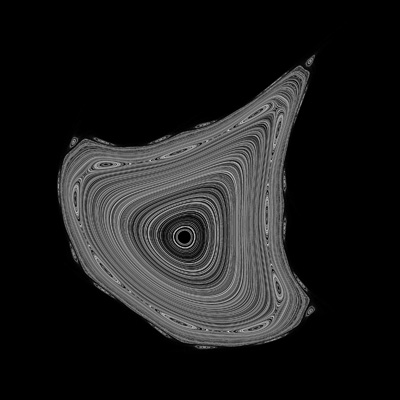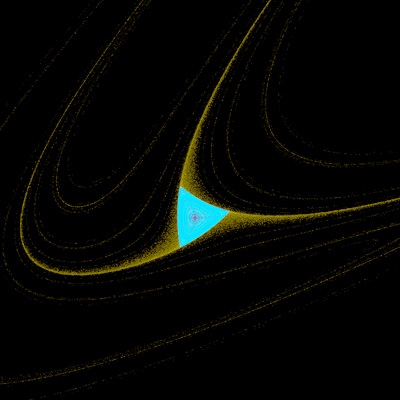# Henon Phase Diagrams

Written by Paul Bourke
January 1991
xn+1 = xn cos(a) - (yn - xn2) sin(a)
yn+1 = xn sin(a) + (yn - xn2) cos(a)a = -10a = 1.57a = 2a = 22a = 1.2a = 1.9a = 2.2a = 1.6